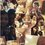# help me

hey guys. help me with this.

two vertical tank in conical shape. both inverted. have their vertices connected by a short horizontal pipe. the larger tank is full of water, has a diameter base of 5 m. the smaller tank is empty, has an altitude of 4 m, has an diameter base of 5 m. if the water is allowed to flow through the connecting pipe until the smaller tank is full. find the total volume of the water in the two tanks and find the altitude of the larger tank.Note by Jeriel Villa
7 years, 5 months ago

This discussion board is a place to discuss our Daily Challenges and the math and science related to those challenges. Explanations are more than just a solution — they should explain the steps and thinking strategies that you used to obtain the solution. Comments should further the discussion of math and science.

When posting on Brilliant:

• Use the emojis to react to an explanation, whether you're congratulating a job well done , or just really confused .
• Ask specific questions about the challenge or the steps in somebody's explanation. Well-posed questions can add a lot to the discussion, but posting "I don't understand!" doesn't help anyone.
• Try to contribute something new to the discussion, whether it is an extension, generalization or other idea related to the challenge.

MarkdownAppears as
*italics* or _italics_ italics
**bold** or __bold__ bold
- bulleted- list
• bulleted
• list
1. numbered2. list
1. numbered
2. list
Note: you must add a full line of space before and after lists for them to show up correctly
paragraph 1paragraph 2

paragraph 1

paragraph 2

[example link](https://brilliant.org)example link
> This is a quote
This is a quote
    # I indented these lines
# 4 spaces, and now they show
# up as a code block.

print "hello world"
# I indented these lines
# 4 spaces, and now they show
# up as a code block.

print "hello world"
MathAppears as
Remember to wrap math in $$ ... $$ or $ ... $ to ensure proper formatting.
2 \times 3 $2 \times 3$
2^{34} $2^{34}$
a_{i-1} $a_{i-1}$
\frac{2}{3} $\frac{2}{3}$
\sqrt{2} $\sqrt{2}$
\sum_{i=1}^3 $\sum_{i=1}^3$
\sin \theta $\sin \theta$
\boxed{123} $\boxed{123}$

Sort by:

mean 222r^2*h/21

- 7 years, 5 months ago

yes

- 7 years, 5 months ago

can you give me the solution on how you solve this?

- 7 years, 5 months ago

the hieght to which water rise will be same i.e 4 m thus the hieght of large tank be consider to be 4m then volume of water will be in 2 tank =222r^2*h/21 =52.38m^3

- 7 years, 5 months ago

so it means that larger tank and smaller tank is equal?

- 7 years, 5 months ago

as the tank connected by pipe so water rise will be same so altitude of large tank be 4m thus applying volume of cone i.e 22r^2h/21, as hieght and diameter is same so ,volume=44r^2h/21 r=d/2,thus r=2.5m ,h=4m , putting values in above equation we get 52.38m^3 if asked in litter then 1m^3=1000ltrs , so 52380ltrs : )

- 7 years, 5 months ago

ok thanks :)

- 7 years, 5 months ago

there diameter is also same

- 7 years, 5 months ago